# Exploration in Reinforcement Learning

How much efforts should be spent on exploration vs exploitation

Everyone is confronted with the same dilemma on a daily basis: should I keep doing what I do, or should I try something else. For example should I go to my preferred restaurant or should I try a new one, should I keep my current job or should I find a new one, etc…

In Reinforcement Learning, this type of decision is called exploitation when you keep doing what you were doing, and exploration when you try something new.

Naturally this raises a question about how much to exploit and how much to explore.

However, there is a problem in exploration which is that we don’t really know what would be the outcome, it could be better than the current situation or it could be worse.

Humans, try to get as much information as possible before making the move, for example before we try a new restaurant we read reviews or ask friends who already tried it. In Reinforcement Learning on the other hand, it is not possible to do that, but there are some techniques that will help figuring out the best strategy.

### Notion of Regret

Logically when we try something new and the result comes unsatisfying we regret our decision. If the new restaurant was bad, we regret going there and we consider whatever amount we paid as a complete loss. So we regret this amount.

As we keep paying for bad decisions the amount of losses grows as well as the level of regret. So it should be a good idea to keep the amount of losses and the level of regret to the minimum.

Can we do that?
The answer is, yes it is possible, at least in RL.

### Regret in Reinforcement Learning

First we need to define the regret in RL. To do so we start by defining the optimal action a* as the action that gives the highest reward.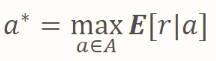Optimal action

So we define the regret L, over the course of T attempts, as the difference between the reward generated by the optimal action a* multiplied by T, and the sum from 1 to T of each reward of an arbitrary action.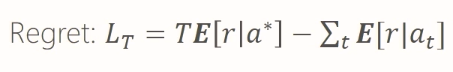It can be proven that as T goes to infinity the regret tends to a lower bound of C . log T.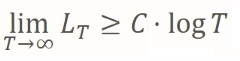### Greedy & Epsilon Greedy

Greedy and Epsilon Greedy exploration methods are fairly easy to understand and to implement, but they suffer from major setback which is they have sub-optimal regret. As a matter of fact the regret of both Greedy and Epsilon Greedy grows linearly by the time.

This is easy to intuitively understand, as the Greedy will lock on one action that happened to have good results at one point of time but it is not in reality the optimal action. So Greedy will keep exploiting this action while ignoring the others which might be better. It Exploits too much.

The Epsilon Greedy on the other hand, explores too much because even when one action seem to be the optimal one, the methods keeps allocating a fixed percentage of the time for exploration, thus missing opportunities and increasing total regret.

Conversely the decaying Epsilon Greedy methods, tries to decrease the percentage dedicated for exploration as time goes by. This can give optimal regret as shown in the graph below.

However the challenge is to be able to perform the right decaying process.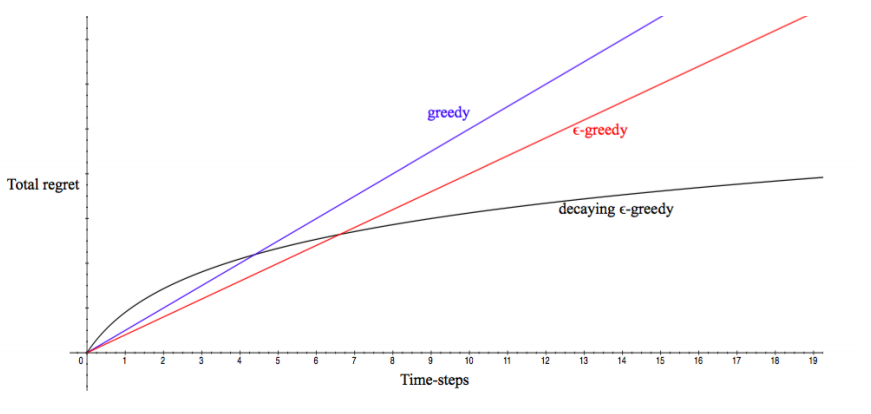### Optimism in Face of Uncertainty

Optimism in face of uncertainty is an approach to enhance the exploration and minimize the total regret.

Suppose we have three slots machine with different probability distribution, that we don’t know exactly, but after few trials we think that we have the following shapes of distribution. Keep in mind that these are drawn out of experience and do not necessarily reflect the truth.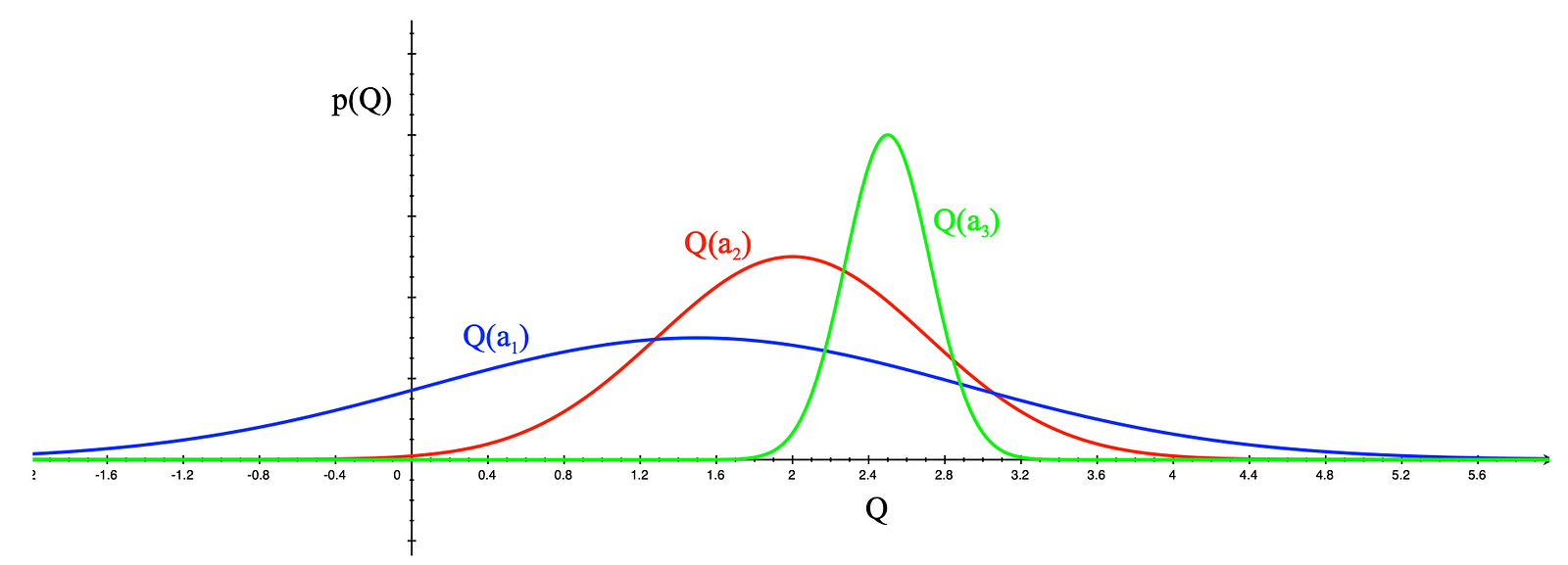The green Q(a3) distribution has rather narrow base because of small interval [1.8 , 3.2], the red Q(a2) has a larger interval [0 , 4], and lastly the blue Q(a1) has the largest interval [-1.8 , 5.2].
So the question is what action should we pick next.

According to the Optimism in Face of Uncertainty principle, we choose the action that present a higher reward than the others even if it has a higher uncertainty. Looking at the graph we easily find that Q(a1) might have a higher reward (5.2) than the others so we chose it even though it has a higher uncertainty (it can be 5.2 as it can be -1.8).

Suppose action a1 was taken and the distributions becomes like the following: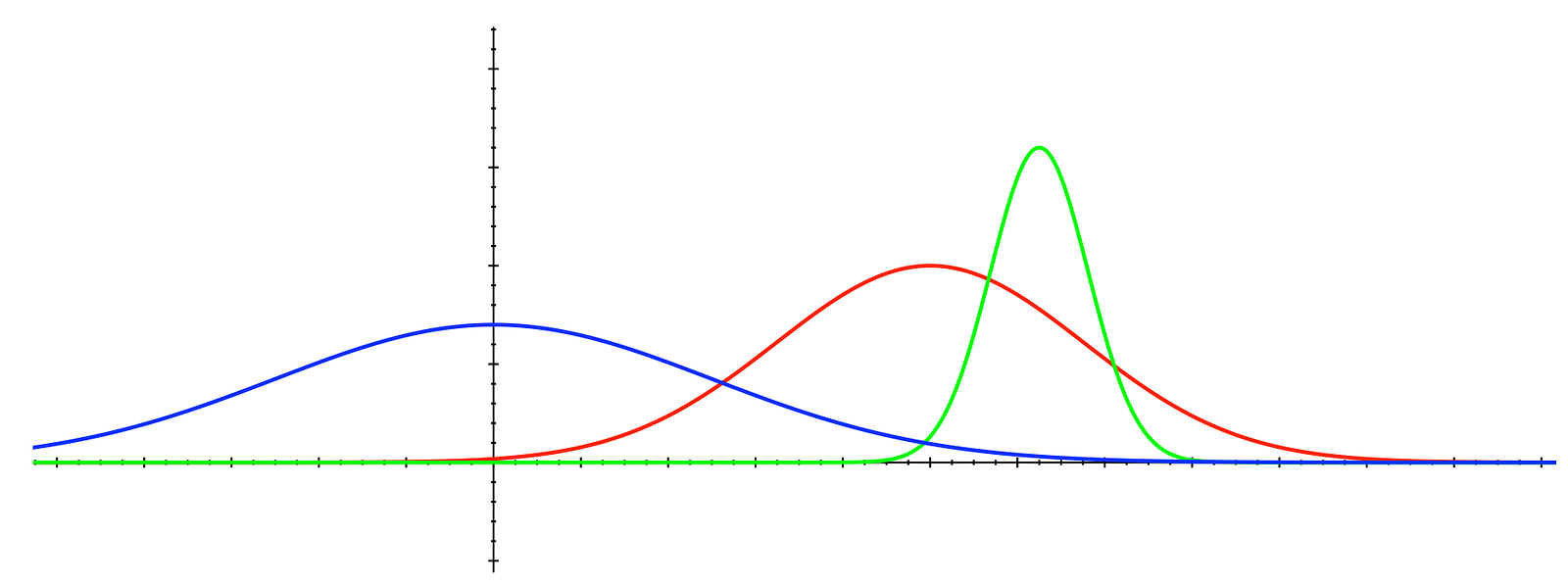We now know that the blue distribution has lesser maximum than the other two. So we are less uncertain about it, and the next action will be chosen from another distribution, the red one for example.

This is the principle, but how will be implemented in reality ?
This is what UCB1 is about.

### UCB1

In the previous paragraph we talked about taking the action which has, potentially, the greatest return even if this is not certain.
However, the question remains is how to find this maximum value?

One way to do that is to estimate it by adding a term called upper confidence bound U?(a) to the Q?(a) for each action then select the action that has the maximum Q?(a) + U?(a).It goes without saying that U?(a) is not constant, but should vary with time and experience.
What we mean is as time goes by and we frequently select action (a), we become more and more certain of what to expect as Q(a), which is by itself an average, so U?(a) should shrink as we become more confident of Q(a).

The formula of U?(a) is as followswhere t is the total number of trials, and N?(a) is the number of times action (a) was selected.
This formula clearly shows that as t increases the numerator also increases but logarithmically (e.g. slowly) while when the action (a) is selected then N?(a) increases by one however the U?(a) decreases sharply.

Here is an example:We can see that for t going from 100 to 1000 and N?(a) is 1, then U?(a) increases very slowly from 2 to 2.45, but when N?(a) increased by 1, U?(a) dropped sharply to 1.73.

So U?(a) is a way to regulate the selection of action (a) based on many times we have already selected this action in the past. In other words, it provides us with more certainty on what reward it might return.

The final algorithm stipulates that for each iteration we compute Q?(a) + U?(a) for all actions and we select the action that has the highest Q?(a) + U?(a) value:This approach of explore vs exploit guarantees to have a logarithmic regret.

IMPORTANT: UCB1 does not assume anything regarding the types of the distribution, even though in the graphs above they look like Gaussian but they can be anything.

#### Gaussian distribution

Let’s assume that we know that the reward distributions are Gaussian :
ℛ(r) = ?(r, μ, σ)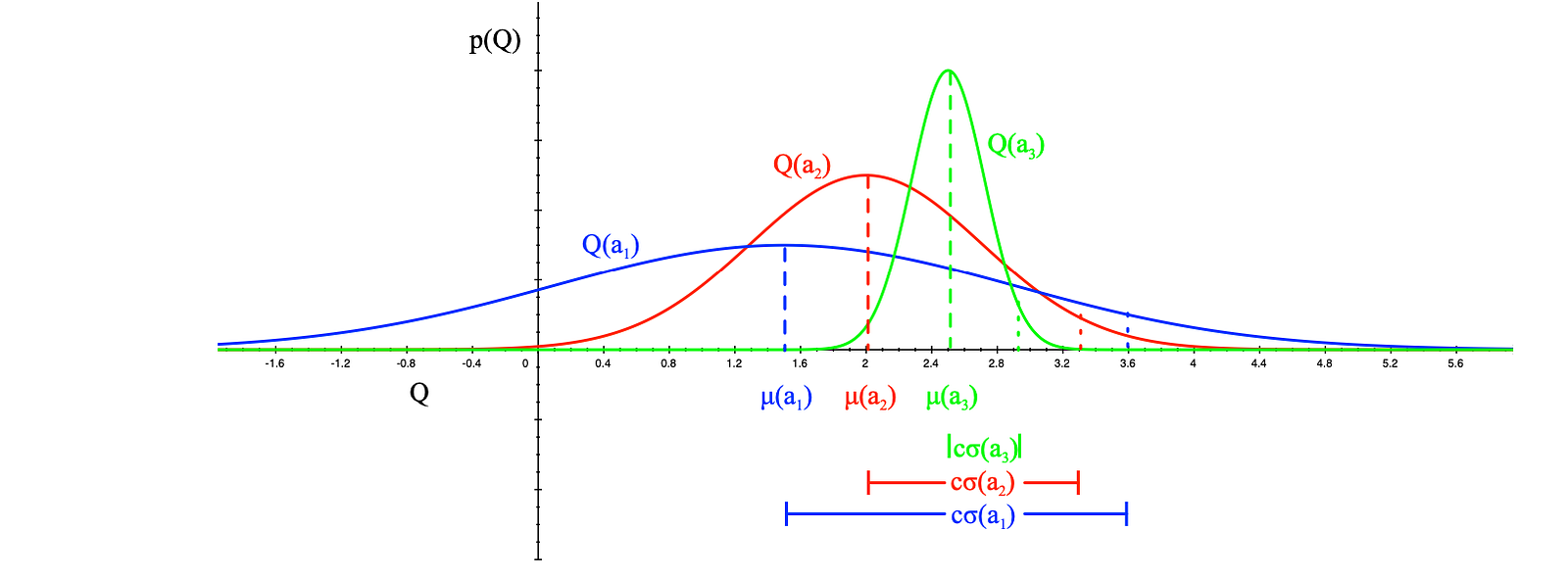The algorithm becomes as picking the action that maximises the standard deviation of Q(a)### Thompson Sampling

Also known as posterior sampling, it is a method that takes samples from each distribution then selects the action from the sample that has the maximum value.
After taking the action and collecting the reward we update the distribution from which we took the action in order to reflect the result that we got.

Of course this needs more explanation than that.

Consider a dice, which we don’t know if it is biased or not, actually we have no information whatsoever about it. So we assume, as a start, that this is fair dice and the probabilities of getting any number are all equal to 1/6.
So the initial belief is that the probability distribution is uniform and equals to 1/6. This is called the Prior distribution and it constitutes a belief or an assumption (it might be a false one) that we have regarding the underlying probability distribution of the dice.
Now we start throwing the dice and collect results, and with each result we update the Prior distribution, to make it reflect the reality of the experiment. The new distribution is now called the Posterior distribution. We keep repeating this process a large number of iterations in which the Posterior at iteration (i) becomes the Prior at iteration (i+1), until we reach a stage where the final Posterior distribution is very close to the real underlying distribution.
For example if, we notice that after a large number of throws we have one number that is preponderant than the others, it means that the distribution can’t be uniform, and most probably the dice is biased.

Now the questions that arises, is how do we update the Prior distribution to obtain the Posterior distribution ?

To answer this question let’s start by defining the Beta distribution:
Beta distribution is a family of continuous probability distributions defined on the interval [0, 1] parametrized by two positive parameters α and β, that determine the shape of the distribution.Formula of the Beta distribution, where C is a constant and ?, β are parameters, and x is between 0 and 1

You can think of the Beta distribution as a family of distributions where each of its member is different than the other according to the values of the parameters ?, β.

The following are some samples of shapes that Beta distribution can have following the values that ?, β can have.

How to sample ?

Sampling consists of randomly getting a value from the distribution, but since the distribution might not be uniform the value that is returned by the sampling is most likely to be from the region where the distribution peaks.

For example, take the third graph in the second row from the above set of graphs. This distribution peaks at the around 0.84. So when sampling from this distribution it is more likely to have value around 0.84, than having a value less than 0.4 for instance.

Now suppose you have multiple actions each with its unknown reward distribution. We use Thompson sampling to discover which action is the best (it maximises the average rewards). We start by assuming a certain distribution such as Beta(1,1) which is a uniform distribution for each action, then we samples those distributions, and select the action that has the highest sample value.
Once the selected action is executed and the reward is collected, the distribution of the selected action is updated.

Let’s take the following example of a multi-armed bandits (slot machines).
The example consists of three multi-armed bandits: blue, red and purple.
Since we don’t know anything about the underlying distribution of these bandits we assume they are uniform. We sample from these distributions and we get the following results: blue = 0.4, red = 0.5, purple = 0.7
It turns out that the purple has the highest sample value, so we pull the arm of the purple machine. Let’s suppose that we didn’t win, so we need to update our belief of the purple distribution by increasing the β parameter by one to become Beta(1, 2) which will give the first graph below.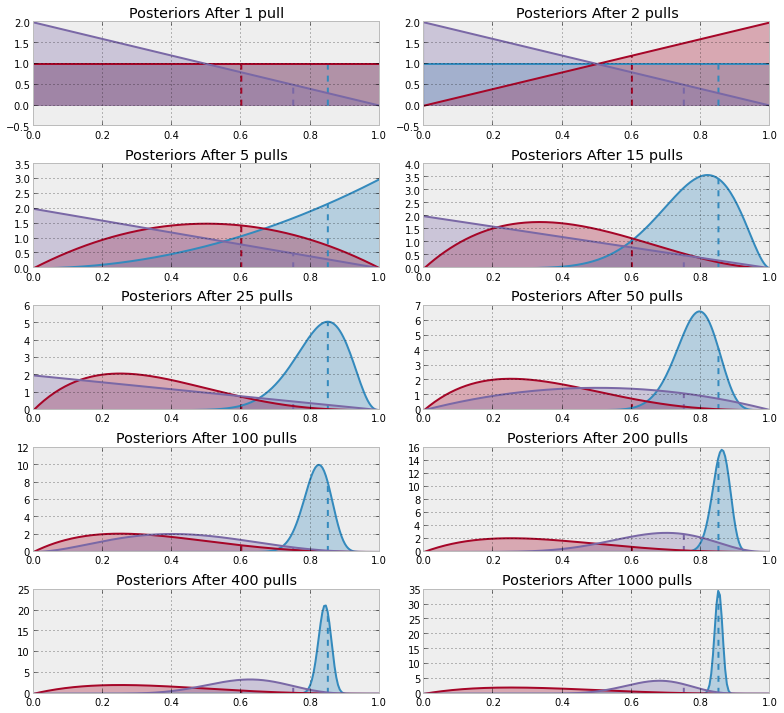We sample again, and this time the red distribution got the highest sample value, so we pull the arm of the red machine and we win. We update the red distribution by increasing ? by one, and we get the second graph (column 2, row 1).
As we continue to sample from all of the distributions we notice that the blue one is getting more wins than the others, so the update gives it more advantage over the rest, and distribution starts to present a peak of likelyhood towards the high values (> 0.8). This means that we are more exploiting the blue machine than exploring the other two. In other words we are more and more convinced that we have found the optimal action.

### Conclusion

This article exposed some of the mostly used exploration techniques employed in Reinforcement Learning. The major take away from it, is to know that exploring actions that have low priority of being optimal is a waste of time and resources. For this reason it is important to use a exploration methods that minimize regrets, so that the learning phase becomes faster and more efficient.

Share